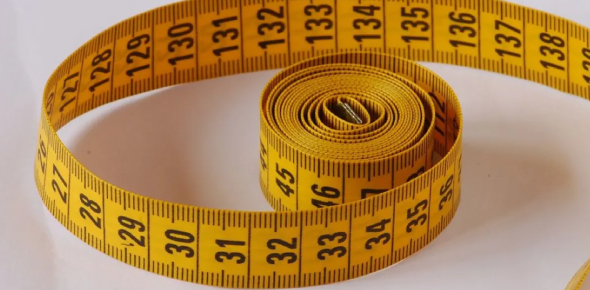# Measurement Test: Math MCQ Quiz!

24 Questions | Total Attempts: 20Settings.

• 1.
The basic unit of mass measurement is ________.
• A.

Kilogram

• B.

Pound

• C.

Ton

• 2.
The appropriate unit of measure for the length of ballpen is the ________.
• A.

Meter

• B.

Centimeter

• C.

Kilometer

• 3.
Which of the following is equal to 3 000 meters?
• A.

3 decimeters

• B.

3 kilometers

• C.

3 centimeters

• 4.
The Math Club members conducted their overnight camping in 2 12days. How many hours did they spend in camping?
• A.

60 hours

• B.

70 hours

• C.

50 hours

• 5.
How many minutes are there in 3 hours?
• A.

90 minutes

• B.

450 minutes

• C.

180 minutes

• 6.
How many days are there in 4 weeks?
• A.

27 days

• B.

14 days

• C.

28 days

• 7.
A writing notebook is about 21 __________
• A.

Kilometer

• B.

Centimeter

• C.

Milliliter

• 8.
Before a baby is born, he stays in his mother’s womb for 9 months. How many weeks is his stay in the womb?
• A.

36

• B.

33

• C.

30

• 9.
Mt. Apo is about 3 kilometers high. About how many meters high is Mt. Apo?
• A.

30 000 m

• B.

3 000 m

• C.

300 m

• 10.
What unit of measure will you use to find the distance from your house to school?
• A.

Decimeter

• B.

Milimiter

• C.

Kilometer

• 11.
4 L of vinegar = _________________ml.
• A.

40ml

• B.

400ml

• C.

4000

• 12.
1. A coffee mug can hold about 160 ______ of coffee.
1. Liters                     c. pints
2. Milliliters                d. quartz
• A.

Pints

• B.

L

• C.

Ml

• 13.
It helps to make the information in a graph clearer and easier to interpret.
• A.

Pie graph

• B.

Title

• C.

Legend

• 14.
It tells what the graph is all about . Example "Favorite Subject"
• A.

Title

• B.

Line graph

• C.

Graph

• 15.
A graph that uses pictures or symbols to present.
• A.

Line graph

• B.

Pictograph

• C.

Bar graph

• 16.
The lowest visited page is __________.
• A.

Andrew

• B.

Emmet

• C.

Michael

• 17.
The 2 highest visited page are_________.
• A.

Emmet and Ying

• B.

Andrew and Ying

• C.

Michael and Emmet

• 18.
What is the Pictograph all about?
• A.

Computer Users

• B.

Penguin Sighting

• C.

Web Pages Visited

• 19.
Read and analyze the problem:        A tennis court is 32 meters long and 25 meters wide. What is its perimeter? What is asked in the problem?
• A.

The distance of the tennis court.

• B.

The perimeter of the tennis court.

• C.

The shape of the tennis court.

• 20.
Read and analyze the problem:        A tennis court is 32 meters long and 25 meters wide. What is its perimeter? What are given?
• A.

32 m; 25 m

• B.

30 m; 32 m

• C.

26 m; 32 m

• 21.
Read and analyze the problem:        A tennis court is 32 meters long and 25 meters wide. What is its perimeter? What operation will be used?
• A.

• B.

Division and Subtraction

• C.

• 22.
Read and analyze the problem:        A tennis court is 32 meters long and 25 meters wide. What is its perimeter? What is the answer? P= ?
• A.

114 m

• B.

14 m

• C.

140m

• 23.
Quarter to 10 is________.
• A.

7:45

• B.

10:45

• C.

9:45

• 24.
5 minutes after 8 o'clock.
• A.

8:05

• B.

9:05

• C.

Option 3

Related TopicsBack to top